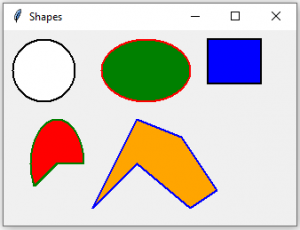# Python Tkinter | Create different shapes using Canvas class

In Tkinter, `Canvas class` is used to create different shapes with the help of some functions which are defined under Canvas class. Any shape that Canvas class creates requires a canvas, so before creating any shapes a Canvas object is required and needs to be packed to the main window.

Canvas Methods for shapes:

Canvas.create_oval(x1, y1, x2, y2, options = …): It is used to create a oval, pieslice and chord.
Canvas.create_rectangle(x1, y1, x2, y2, options = …): It is used to create rectangle and square.
Canvas.create_arc(x1, y1, x2, y2, options = …) This is used to create an arc.
Canvas.create_polygon(coordinates, options = …) THis is used to create any valid shapes.

We are using a class to show the working of functions that helps to creates different shapes.

Class parameters –

Data members used: master, canvas

Member functions used: `create()` method

Widgets used: Canvas

Tkinter method used:
Canvas.create_oval()
Canvas.create_rectangle()
Canvas.create_arc()
Canvas.create_polygon()
pack()
title()
geometry()

Below is the Python code –

 `# Imports each and every method and class ` `# of module tkinter and tkinter.ttk ` `from` `tkinter ``import` `*` `from` `tkinter.ttk ``import` `*` `class` `Shape: ` `    ``def` `__init__(``self``, master ``=` `None``): ` `        ``self``.master ``=` `master ` `         `  `        ``# Calls create method of class Shape ` `        ``self``.create() ` `     `  `    ``def` `create(``self``): ` `         `  `        ``# Creates a object of class canvas ` `        ``# with the help of this we can create different shapes ` `        ``self``.canvas ``=` `Canvas(``self``.master) ` ` `  `        ``# Creates a circle of diameter 80 ` `        ``self``.canvas.create_oval(``10``, ``10``, ``80``, ``80``,  ` `                            ``outline ``=` `"black"``, fill ``=` `"white"``, ` `                            ``width ``=` `2``) ` `         `  `        ``# Creates an ellipse with horizontal diameter ` `        ``# of 210 and vertical diameter of 80 ` `        ``self``.canvas.create_oval(``110``, ``10``, ``210``, ``80``, ` `                            ``outline ``=` `"red"``, fill ``=` `"green"``, ` `                            ``width ``=` `2``) ` `         `  `        ``# Creates a rectangle of 50x60 (heightxwidth) ` `        ``self``.canvas.create_rectangle(``230``, ``10``, ``290``, ``60``, ` `                                ``outline ``=` `"black"``, fill ``=` `"blue"``, ` `                                ``width ``=` `2``) ` `         `  `        ``# Creates an arc of 210 deg ` `        ``self``.canvas.create_arc(``30``, ``200``, ``90``, ``100``, start ``=` `0``, ` `                          ``extent ``=` `210``, outline ``=` `"green"``, ` `                          ``fill ``=` `"red"``, width ``=` `2``) ` `         `  `        ``points ``=` `[``150``, ``100``, ``200``, ``120``, ``240``, ``180``, ` `                  ``210``, ``200``, ``150``, ``150``, ``100``, ``200``] ` `         `  `        ``# Creates a polygon ` `        ``self``.canvas.create_polygon(points, outline ``=` `"blue"``, ` `                              ``fill ``=` `"orange"``, width ``=` `2``) ` `        ``# Pack the canvas to the main window and make it expandable ` `        ``self``.canvas.pack(fill ``=` `BOTH, expand ``=` `1``) ` ` `  `if` `__name__ ``=``=` `"__main__"``: ` `     `  `    ``# object of class Tk, resposible for creating ` `    ``# a tkinter toplevel window ` `    ``master ``=` `Tk() ` `    ``shape ``=` `Shape(master) ` ` `  `    ``# Sets the title to Shapes ` `    ``master.title(``"Shapes"``) ` ` `  `    ``# Sets the geometry and position ` `    ``# of window on the screen ` `    ``master.geometry(``"330x220 + 300 + 300"``) ` ` `  `    ``# Infnite loop breaks only by interrupt ` `    ``mainloop() `

Output:My Personal Notes arrow_drop_upCheck out this Author's contributed articles.

If you like GeeksforGeeks and would like to contribute, you can also write an article using contribute.geeksforgeeks.org or mail your article to contribute@geeksforgeeks.org. See your article appearing on the GeeksforGeeks main page and help other Geeks.

Please Improve this article if you find anything incorrect by clicking on the "Improve Article" button below.

Article Tags :

Be the First to upvote.

Please write to us at contribute@geeksforgeeks.org to report any issue with the above content.